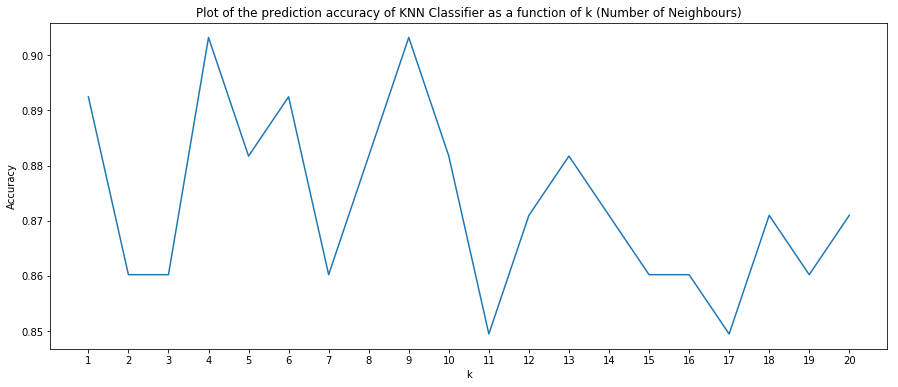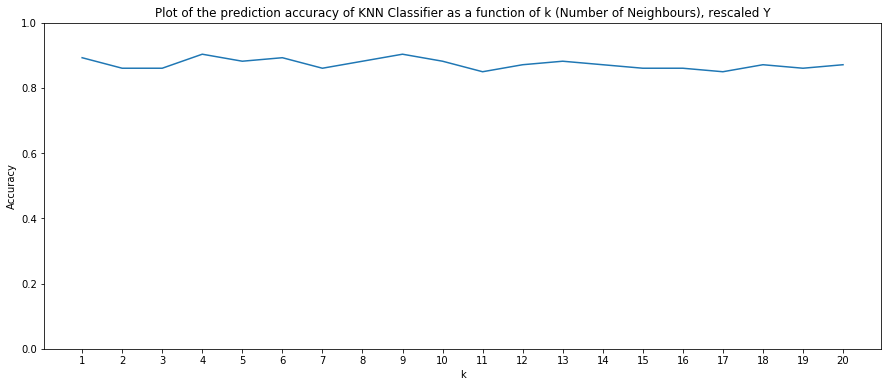### Objective: Predict if a mail is spam or not¶

Data Description:

Refer: https://archive.ics.uci.edu/ml/datasets/spambase for detailed data description

• Number of Observations: 4601 (1813 Spam = 39.4%)
• Number of Attributes: 58 (57 continuous, 1 nominal class label)

Attributes

• 48 continuous real [0,100] attributes of type word_freq_WORD = percentage of words in the e-mail that match WORD, i.e. 100 * (number of times the WORD appears in the e-mail) / total number of words in e-mail. A "word" in this case is any string of alphanumeric characters bounded by non-alphanumeric characters or end-of-string.

• 6 continuous real [0,100] attributes of type char_freq_CHAR] = percentage of characters in the e-mail that match CHAR, i.e. 100 * (number of CHAR occurences) / total characters in e-mail

• 1 continuous real [1,...] attribute of type capital_run_length_average = average length of uninterrupted sequences of capital letters

• 1 continuous integer [1,...] attribute of type capital_run_length_longest = length of longest uninterrupted sequence of capital letters

• 1 continuous integer [1,...] attribute of type capital_run_length_total = sum of length of uninterrupted sequences of capital letters = total number of capital letters in the e-mail

• 1 nominal {0,1} class attribute of type spam = denotes whether the e-mail was considered spam (1) or not (0), i.e. unsolicited commercial e-mail.

K-Nearest Neighbors Classifier

In this classification technique, the distance between the new point (unlabelled) and all the other labelled points is computed. The K-closest labelled points are obtained and the majority vote of their classes is the class assigned to the unlabelled point. All ties are broken arbitrarily.

Data used for this implementation is available at Github Link

### k-NN Algorithm for k = 1, 2 ... 20¶

In :
## Imports and Loading Data

import numpy as np
import matplotlib.pyplot as plt
import operator
#import scipy
from scipy.special import expit
%matplotlib inline

X_train = np.genfromtxt('hw2-data/X_train.csv', delimiter=',')
y_train = np.genfromtxt('hw2-data/y_train.csv')

X_test = np.genfromtxt('hw2-data/X_test.csv', delimiter=',')
y_test = np.genfromtxt('hw2-data/y_test.csv')

In :
def euclidean_distance(vector1, vector2):
return np.sqrt(np.sum(np.power(vector1-vector2, 2)))
def absolute_distance(vector1, vector2):
return np.sum(np.absolute(vector1-vector2))

In :
def get_neighbours(X_train, X_test_instance, k):
distances = []
neighbors = []
for i in xrange(0, X_train.shape):
dist = absolute_distance(X_train[i], X_test_instance)
distances.append((i, dist))
distances.sort(key=operator.itemgetter(1))
for x in xrange(k):
#print distances[x]
neighbors.append(distances[x])
return neighbors

In :
def predictkNNClass(output, y_train):
for i in xrange(len(output)):
#         print output[i], y_train[output[i]]
else:

In :
def kNN_test(X_train, X_test, Y_train, Y_test, k):
output_classes = []
for i in xrange(0, X_test.shape):
output = get_neighbours(X_train, X_test[i], k)
predictedClass = predictkNNClass(output, Y_train)
output_classes.append(predictedClass)
return output_classes

In :
def prediction_accuracy(predicted_labels, original_labels):
count = 0
for i in xrange(len(predicted_labels)):
if predicted_labels[i] == original_labels[i]:
count += 1
#print count, len(predicted_labels)
return float(count)/len(predicted_labels)

In :
predicted_classes = {}
final_accuracies = {}
for k in xrange(1, 21):
predicted_classes[k] = kNN_test(X_train, X_test, y_train, y_test, k)
final_accuracies[k] = prediction_accuracy(predicted_classes[k], y_test)


### Showing Prediction Accuracy as a function of K¶

In :
plt.figure(figsize=(15, 6))
plt.plot(final_accuracies.keys(), final_accuracies.values())
plt.xticks(final_accuracies.keys())
plt.xlabel("k")
plt.ylabel("Accuracy")
plt.title("Plot of the prediction accuracy of KNN Classifier as a function of k (Number of Neighbours)")
plt.show()### Showing Prediction Accuracy as a function of K (adjusted y-scale to between 0-1)¶

In :
plt.figure(figsize=(15, 6))
plt.plot(final_accuracies.keys(), final_accuracies.values())
plt.ylim([0, 1])
plt.xticks(final_accuracies.keys())
plt.xlabel("k")
plt.ylabel("Accuracy")
plt.title("Plot of the prediction accuracy of KNN Classifier as a function of k (Number of Neighbours), rescaled Y")
plt.show()